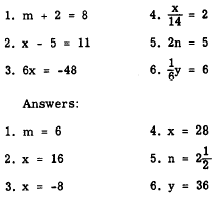Solving linear equationsCustom SearchSOLVING LINEAR EQUATIONS Solving a linear equation in one variable means finding the value of the variable that makes the equation true. For example, 11 is the SOLUTION of x - 7 = 4, since 11 - 7 = 4. The number 11 is said to SATISFY the equation. Basically, the operation used in solving equations is to manipulate both members, by addition, subtraction, multiplication, or division until the value of the variable becomes apparent. This manipulation may be accomplished in a straightforward manner by use of the axioms outlined in chapter 3 of this course. These axioms may be summed up in the following rule: If both members of an equation are increased, decreased, multiplied, or divided by the same number, or by equal numbers, the results will be equal. (Division by zero is excluded.) As mentioned earlier, an equation may be compared to a balance. What is done to one member must also be done to the other to maintain a balance. An equation must always be kept in balance or the equality is lost. We use the above rule to remove or adjust terms and coefficients until the value of the variable is discovered. Some examples of equations solved by means of the four operations mentioned in the rule are given in the following paragraphs. ADDITION Find the value of x in the equation         x-3=12 As in any equation, we must isolate the variable on either the right or left side. In this problem, we leave the variable on the left and perform the following steps: 1. Add 3 to both members of the equation, as follows:         x - 3 + 3 = 12 + 3 In effect, we are "undoing" the subtraction indicated by the expression x - 3, for the purpose of isolating x in the left member.  2. Combining terms, we have         x = 15 SUBTRACTION Find the value of x in the equation         x + 14 = 24 1. Subtract 14 from each member. In effect, this undoes the addition indicated in the expression x + 14.         x + 14 - 14 = 24 - 14 2. Combining terms, we have         x = 10 MULTIPLICATION Find the value of y in the equation         y/5 = 10 1. The only way to remove the 5 so that the y can be isolated is to undo the indicated division. Thus we use the inverse of division, which is multiplication. Multiplying both members by 5, we have the following:         5(y/5) = 5(10) 2. Performing the indicated multiplications, we have          y = 50 DIVISION Find the value of x in the equation 3x = 15 .  1. The multiplier 3 may be removed from the x by dividing the left member by 3. This must be balanced by dividing the right member by 3 also, as follows: 2. Performing the indicated divisions, we have          x=5  Practice problems. Solve the following equations:Integrated Publishing, Inc. - A (SDVOSB) Service Disabled Veteran Owned Small Business